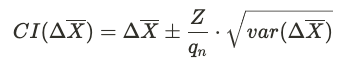# Sequential Testing

## What is Sequential Testing?​

Traditional A/B testing best practices dictate that the readout of experiment metrics should occur only once, when the target sample size of the experiment has been reached. Continuous monitoring for the purpose of decision making results in inflated false positive rates (a.k.a. the peeking problem), much higher than expected based on the significance level selected for the test.

This is because p-values fluctuate and are likely to drop in and out of significance just by random chance, even when there is no real effect. Continuous monitoring introduces selection bias in the date we pick for the readout: Selectively choosing a date based on the observed results is essentially cherry-picking a stat-sig result that would never be observed if the data were to be analyzed only over the entire, pre-determined duration of the experiment. This increases the false positive rate (observing an experimental effect when there is none).

In Sequential Testing, the p-values for each preliminary analysis window are adjusted to compensate for the increased false positive rate associated with peeking. The goal is to enable early decision making when there's sufficient evidence while limiting the risk of false positives. While peeking is typically discouraged, regular monitoring of experiments with sequential testing is particularly valuable in some cases. For example:

• Unexpected regressions: Sometimes experiments have bugs or unintended consequences that severely impact key metrics. Sequential testing helps identify these regressions early and distinguishes significant effects from random fluctuations.
• Opportunity cost: This arises when a significant loss may be incurred by delaying the experiment decision, such as launching a new feature ahead of a major event or fixing a bug. If sequential testing shows an improvement in the key metrics, an early decision could be made. But use caution: An early stat-sig result for certain metrics doesn't guarantee sufficient power to detect regressions in other metrics. Limit this approach to cases where only a small number of metrics are relevant to the decision.

## Quick Guide: Interpreting Sequential Testing Results​

Click on Edit at the top of the metrics section in Pulse to toggle Sequential Testing on/off.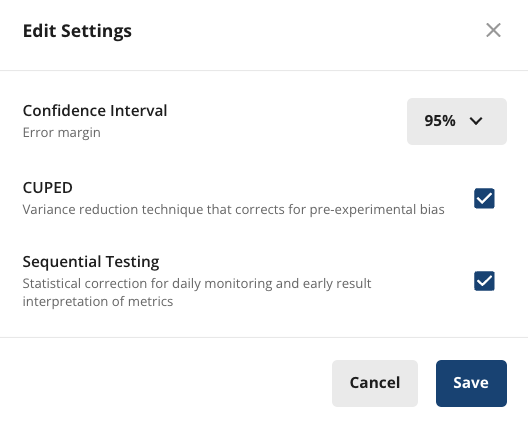When enabled, an adjustment is automatically applied to results calculated before the target completion date of the experiment.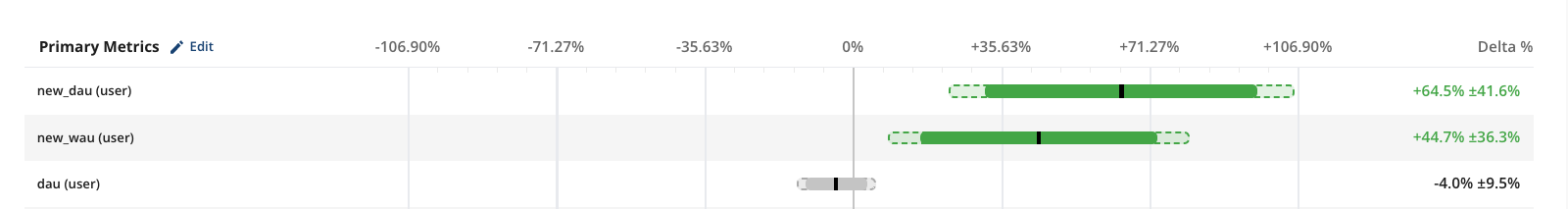The dashed line represents the expanded confidence interval resulting from the adjustment. The solid bar is the standard confidence interval computed without any adjustments. If the adjusted confidence interval overlaps with zero, this means the metric delta is not stat-sig at the moment, and the experiment should continue its course as planned.

Hover over a metric and click View Details to review the progression of the sequential test.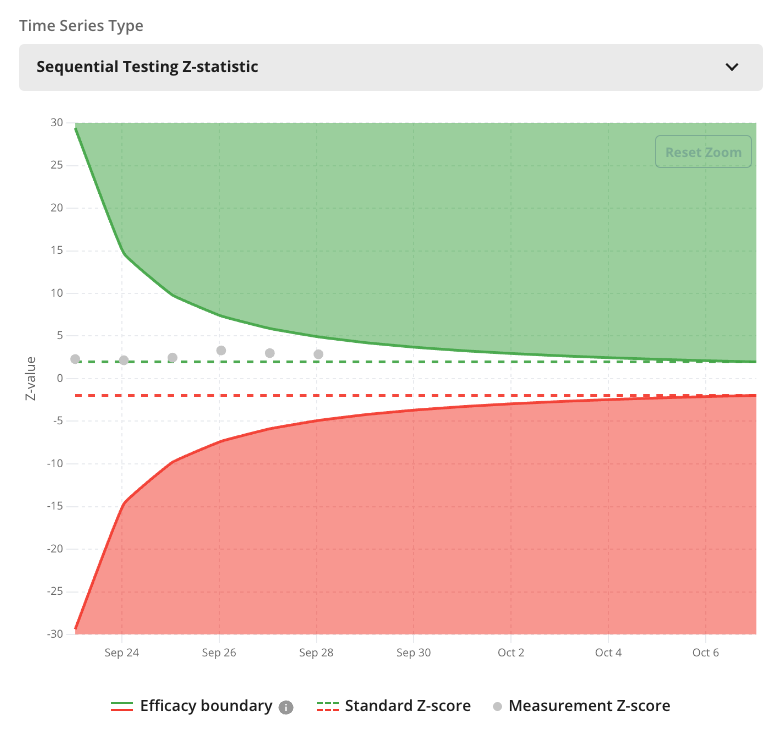The Sequential Testing Z-Statistic time series contains the following information for a metric:

• Efficacy Boundaries (solid red and green curves): The thresholds for positive and negative statistical significance. These start out high, signifying the increased confidence needed for making an early decision. When the target duration is reached, they converge to the standard Z-score for the selected significance level (dashed lines).
• Measurement Z-score (dots): These are the Z-scores computed each day for the test vs. control comparison. A Z-score higher than the upper efficacy boundary is stat-sig positive. One lower than the bottom boundary is stat-sig negative.

## Statsig's Implementation of Sequential Testing​

We use an adjustment factor qn that's determined by the number of days n the experiment has been running: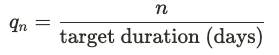When the target duration is reached, qn = 1 and no more adjustments are applied. This method has 2 benefits:

• Simplicity: The calculation of the adjustment factor is easy to understand. It also satisfies the intuitive expectation that the significance threshold be higher early on.
• Power: When the target duration is reached, the efficacy boundary converges with the standard Z-score for the selected significance level. Therefore, there is no loss in statistical power when doing a metrics readout at the conclusion of the pre-determined experiment duration. We selected this approach because we believe the primary value of sequential testing is to provide higher confidence when making early decisions based on unexpected metric movements, such as ending an experiment early due to a large regression. However, in most cases it's best make a decision based on the complete set of relevant metrics at the end of the experiment, without any adjustments that reduce power.

### Efficacy Boundary and Z-score Calculation​

On any given day n, the efficacy boundary is given by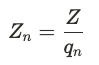where Z is the standard Z-score for the desired significance level (e.g.: 1.96 for two-sided test with $\alpha$ = 0.05). This determines the Z-score threshold for statistical significance on day n.

The Z-statistic for a metric comparison (ZX) is computed in the standard way: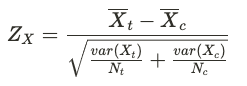A metric is stat-sig when the calculated Z-score falls outside of the efficacy boundary. Specifically:

• ZX > Zn is stat-sig positive even after Sequential Testing adjustment
• ZX < -Zn is stat-sig negative even after Sequential Testing adjustment
• Zn > ZX > Z or -Zn < ZX < -Z is not stat-sig with the adjustment, but would be stat-sig without it. These are possible false positive that can be avoided with Sequential Testing
• Z > ZX > -Z is not stat-sig

### Adjusted p-values and Confidence Intervals​

The p-value calculation for day n is similar to the standard calculation, but with the Z-score scaled by a factor of qn. This leads to higher p-values, meaning the bar for statistical significance is higher.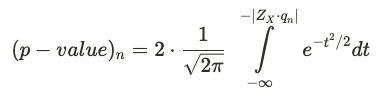Similarly, the confidence intervals (CI) are adjusted by a factor of 1/qn, leading to larger CIs when qn < 1.